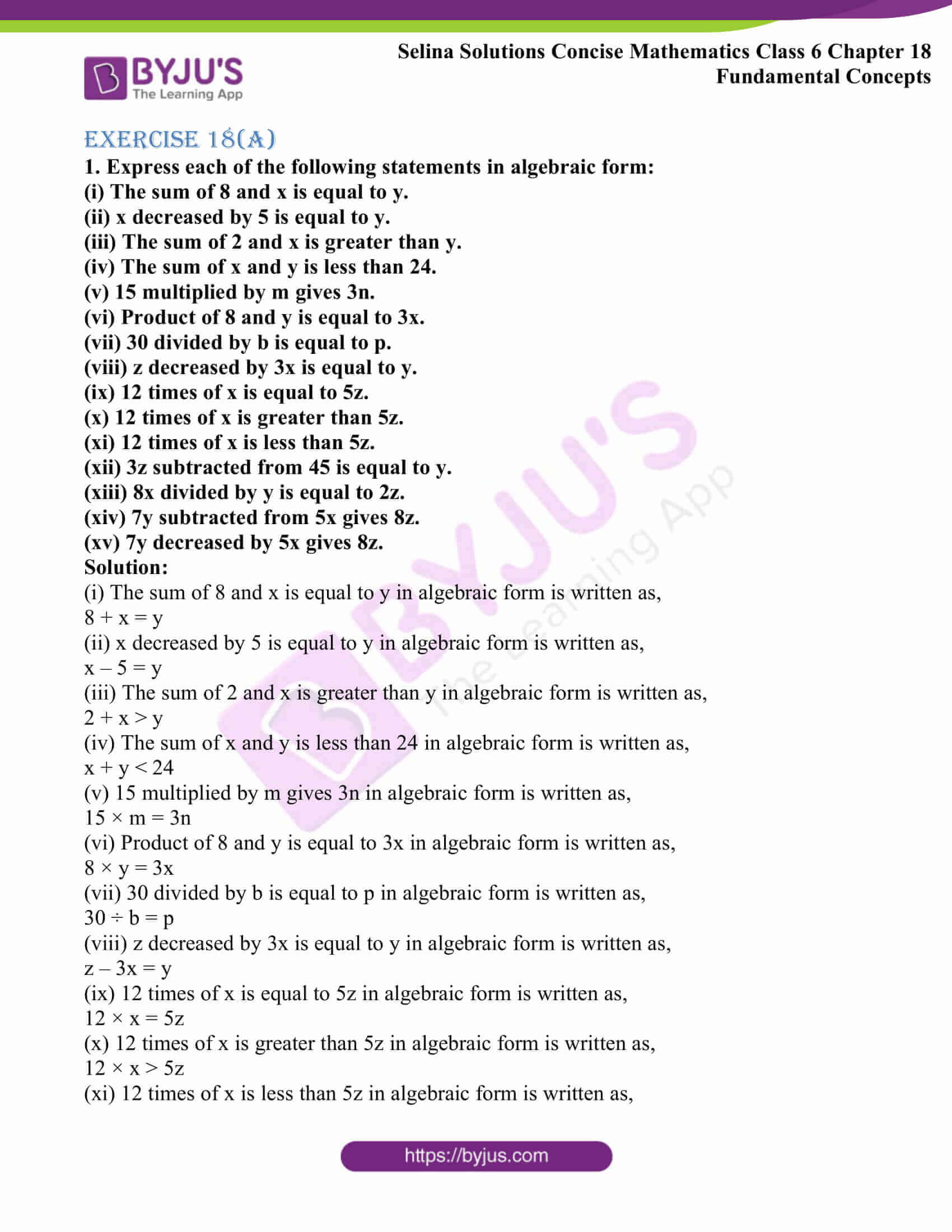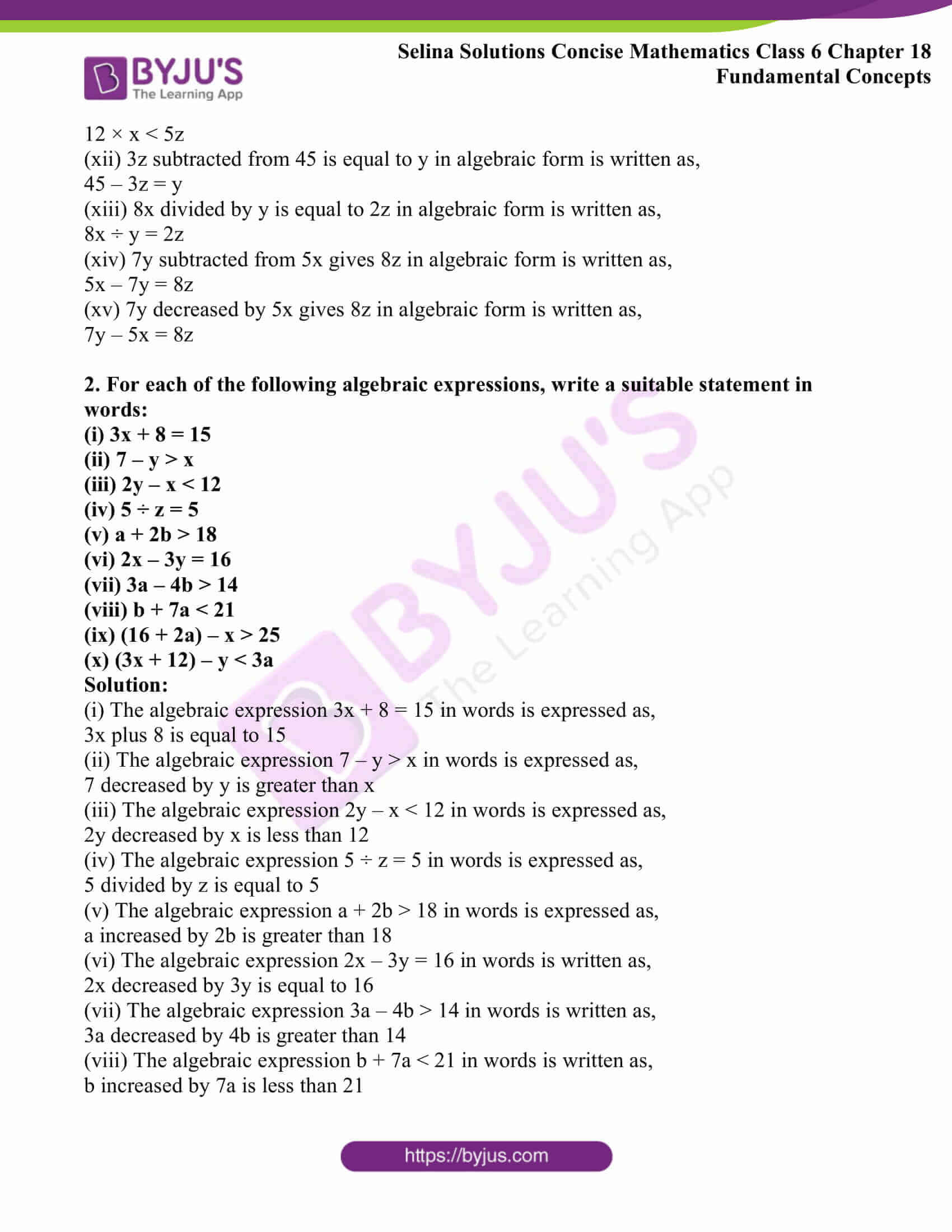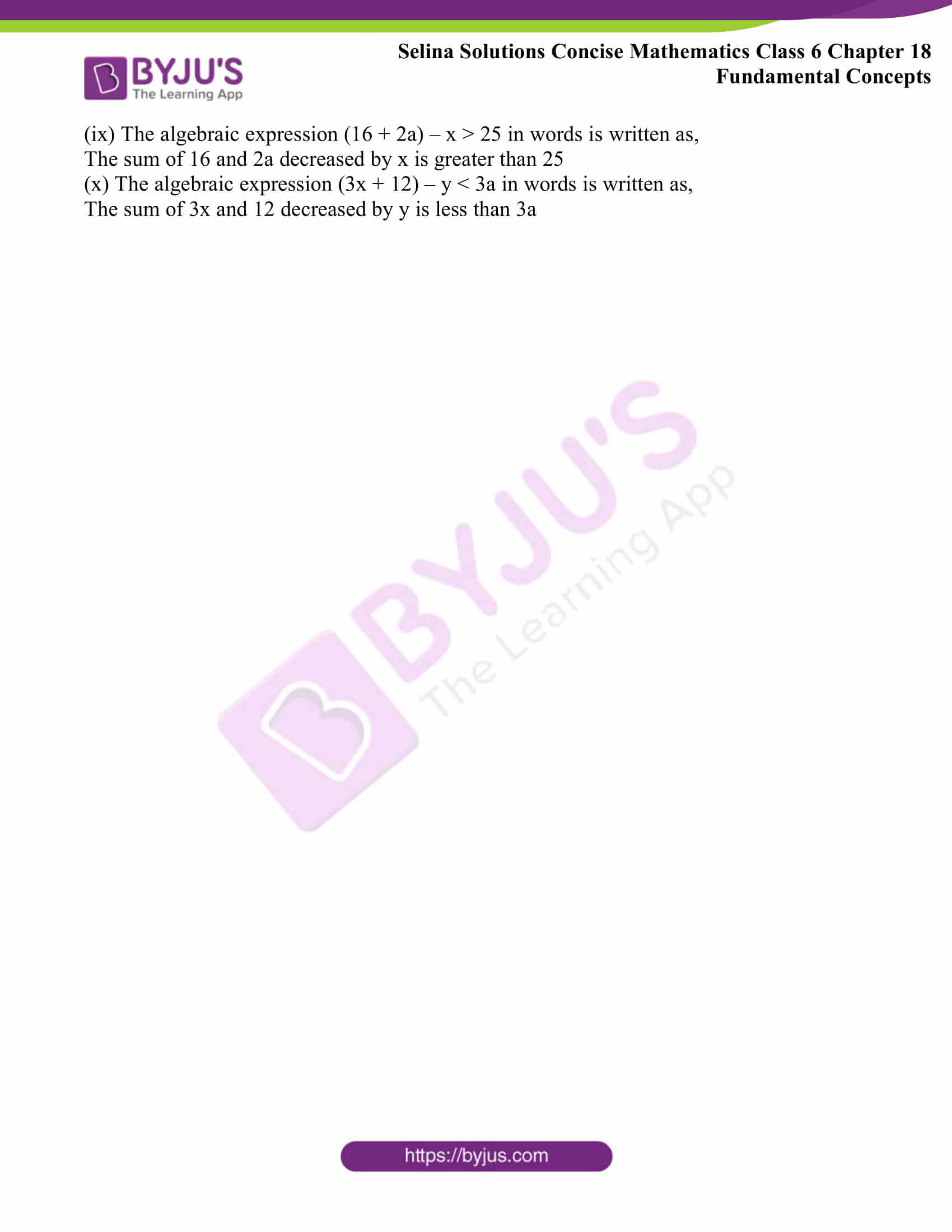Selina Solutions Concise Mathematics Class 6 Chapter 18 Fundamental Concepts Exercise 18(A) provides in-depth knowledge of the concepts, relying on algebraic expressions. The solutions contain important tricks, which can be followed in solving the problems with ease. The main objective of preparing solutions PDF is to improve time management skills, along with problem solving abilities among students. To score well in the annual exam, Selina Solutions Concise Mathematics Class 6 Chapter 18 Fundamental Concepts Exercise 18(A) PDF links, which are given here for free download.

## Selina Solutions Concise Mathematics Class 6 Chapter 18: Fundamental Concepts Exercise 18(A) Download PDF### Access Selina Solutions Concise Mathematics Class 6 Chapter 18: Fundamental Concepts Exercise 18(A)

Exercise 18(A)

1. Express each of the following statements in algebraic form:

(i) The sum of 8 and x is equal to y.

(ii) x decreased by 5 is equal to y.

(iii) The sum of 2 and x is greater than y.

(iv) The sum of x and y is less than 24.

(v) 15 multiplied by m gives 3n.

(vi) Product of 8 and y is equal to 3x.

(vii) 30 divided by b is equal to p.

(viii) z decreased by 3x is equal to y.

(ix) 12 times of x is equal to 5z.

(x) 12 times of x is greater than 5z.

(xi) 12 times of x is less than 5z.

(xii) 3z subtracted from 45 is equal to y.

(xiii) 8x divided by y is equal to 2z.

(xiv) 7y subtracted from 5x gives 8z.

(xv) 7y decreased by 5x gives 8z.

Solution:

(i) The sum of 8 and x is equal to y in algebraic form is written as,

8 + x = y

(ii) x decreased by 5 is equal to y in algebraic form is written as,

x – 5 = y

(iii) The sum of 2 and x is greater than y in algebraic form is written as,

2 + x > y

(iv) The sum of x and y is less than 24 in algebraic form is written as,

x + y < 24

(v) 15 multiplied by m gives 3n in algebraic form is written as,

15 × m = 3n

(vi) Product of 8 and y is equal to 3x in algebraic form is written as,

8 × y = 3x

(vii) 30 divided by b is equal to p in algebraic form is written as,

30 ÷ b = p

(viii) z decreased by 3x is equal to y in algebraic form is written as,

z – 3x = y

(ix) 12 times of x is equal to 5z in algebraic form is written as,

12 × x = 5z

(x) 12 times of x is greater than 5z in algebraic form is written as,

12 × x > 5z

(xi) 12 times of x is less than 5z in algebraic form is written as,

12 × x < 5z

(xii) 3z subtracted from 45 is equal to y in algebraic form is written as,

45 – 3z = y

(xiii) 8x divided by y is equal to 2z in algebraic form is written as,

8x ÷ y = 2z

(xiv) 7y subtracted from 5x gives 8z in algebraic form is written as,

5x – 7y = 8z

(xv) 7y decreased by 5x gives 8z in algebraic form is written as,

7y – 5x = 8z

2. For each of the following algebraic expressions, write a suitable statement in words:

(i) 3x + 8 = 15

(ii) 7 – y > x

(iii) 2y – x < 12

(iv) 5 ÷ z = 5

(v) a + 2b > 18

(vi) 2x – 3y = 16

(vii) 3a – 4b > 14

(viii) b + 7a < 21

(ix) (16 + 2a) – x > 25

(x) (3x + 12) – y < 3a

Solution:

(i) The algebraic expression 3x + 8 = 15 in words is expressed as,

3x plus 8 is equal to 15

(ii) The algebraic expression 7 – y > x in words is expressed as,

7 decreased by y is greater than x

(iii) The algebraic expression 2y – x < 12 in words is expressed as,

2y decreased by x is less than 12

(iv) The algebraic expression 5 ÷ z = 5 in words is expressed as,

5 divided by z is equal to 5

(v) The algebraic expression a + 2b > 18 in words is expressed as,

a increased by 2b is greater than 18

(vi) The algebraic expression 2x – 3y = 16 in words is written as,

2x decreased by 3y is equal to 16

(vii) The algebraic expression 3a – 4b > 14 in words is written as,

3a decreased by 4b is greater than 14

(viii) The algebraic expression b + 7a < 21 in words is written as,

b increased by 7a is less than 21

(ix) The algebraic expression (16 + 2a) – x > 25 in words is written as,

The sum of 16 and 2a decreased by x is greater than 25

(x) The algebraic expression (3x + 12) – y < 3a in words is written as,

The sum of 3x and 12 decreased by y is less than 3a